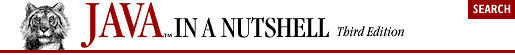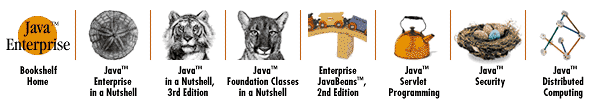home | O'Reilly's CD bookshelfs | FreeBSD | Linux | Cisco | Cisco Exam## 3.6. Abstract Classes and Methods

In Example 3-4, we declared our Circle class to be part of a package named shapes. Suppose we plan to implement a number of shape classes: Rectangle, Square, Ellipse, Triangle, and so on. We can give these shape classes our two basic area() and circumference() methods. Now, to make it easy to work with an array of shapes, it would be helpful if all our shape classes had a common superclass, Shape. If we structure our class hierarchy this way, every shape object, regardless of the actual type of shape it represents, can be assigned to variables, fields, or array elements of type Shape. We want the Shape class to encapsulate whatever features all our shapes have in common (e.g., the area() and circumference() methods). But our generic Shape class doesn't represent any real kind of shape, so it cannot define useful implementations of the methods. Java handles this situation with abstract methods.

Java lets us define a method without implementing it by declaring the method with the abstract modifier. An abstract method has no body; it simply has a signature definition followed by a semicolon. Here are the rules about abstract methods and the abstract classes that contain them:

 An abstract method in Java is something like a pure virtual function in C++ (i.e., a virtual function that is declared = 0). In C++, a class that contains a pure virtual function is called an abstract class and cannot be instantiated. The same is true of Java classes that contain abstract methods.

• Any class with an abstract method is automatically abstract itself and must be declared as such.

• An abstract class cannot be instantiated.

• A subclass of an abstract class can be instantiated only if it overrides each of the abstract methods of its superclass and provides an implementation (i.e., a method body) for all of them. Such a class is often called a concrete subclass, to emphasize the fact that it is not abstract.

• If a subclass of an abstract class does not implement all the abstract methods it inherits, that subclass is itself abstract.

• static, private, and final methods cannot be abstract, since these types of methods cannot be overridden by a subclass. Similarly, a final class cannot contain any abstract methods.

• A class can be declared abstract even if it does not actually have any abstract methods. Declaring such a class abstract indicates that the implementation is somehow incomplete and is meant to serve as a superclass for one or more subclasses that will complete the implementation. Such a class cannot be instantiated.

There is an important feature of the rules of abstract methods. If we define the Shape class to have abstractarea() and circumference() methods, any subclass of Shape is required to provide implementations of these methods so it can be instantiated. In other words, every Shape object is guaranteed to have implementations of these methods defined. Example 3-5 shows how this might work. It defines an abstractShape class and two concrete subclasses of it.

#### Example 3-5. An Abstract Class and Concrete Subclasses

```public abstract class Shape {
public abstract double area();            // Abstract methods: note
public abstract double circumference();   // semicolon instead of body.
}

class Circle extends Shape {
public static final double PI = 3.14159265358979323846;
protected double r;                              // Instance data
public Circle(double r) { this.r = r; }          // Constructor
public double getRadius() { return r; }          // Accessor
public double area() { return PI*r*r; }          // Implementations of
public double circumference() { return 2*PI*r; } // abstract methods.
}

class Rectangle extends Shape {
protected double w, h;                               // Instance data
public Rectangle(double w, double h) {               // Constructor
this.w = w;  this.h = h;
}
public double getWidth() { return w; }               // Accessor method
public double getHeight() { return h; }              // Another accessor
public double area() { return w*h; }                 // Implementations of
public double circumference() { return 2*(w + h); }  // abstract methods.
}```

Each abstract method in Shape has a semicolon right after its parentheses. There are no curly braces, and no method body is defined. Using the classes defined in Example 3-5, we can now write code like this:

```Shape[] shapes = new Shape;          // Create an array to hold shapes
shapes = new Circle(2.0);            // Fill in the array
shapes = new Rectangle(1.0, 3.0);
shapes = new Rectangle(4.0, 2.0);

double total_area = 0;
for(int i = 0; i < shapes.length; i++)
total_area += shapes[i].area();     // Compute the area of the shapes```

There are two important points to notice here:

• Subclasses of Shape can be assigned to elements of an array of Shape. No cast is necessary. This is another example of a widening reference type conversion (discussed in Chapter 2, "Java Syntax from the Ground Up").

• You can invoke the area() and circumference() methods for any Shape object, even though the Shape class does not define a body for these methods. When you do this, the method to be invoked is found using dynamic method lookup, so the area of a circle is computed using the method defined by Circle, and the area of a rectangle is computed using the method defined by Rectangle.